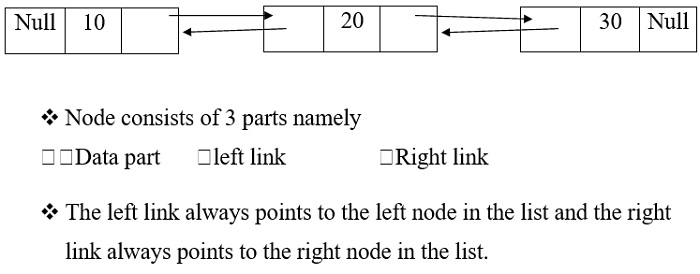# C program to insert a node at any position using double linked list

Linked lists use dynamic memory allocation and are collection of nodes.

Nodes have two parts which are data and link.

The types of linked lists in C programming language are as follows −

• Single / Singly linked lists.
• Double / Doubly linked lists.

The diagram given below depicts the representation of double linked list.## Example

Following is the C program to insert a node at any position using double linked list

Live Demo

#include <stdio.h>
#include <stdlib.h>
struct node {
int num;
struct node * preptr;
struct node * nextptr;
}*stnode, *ennode;
void DlListcreation(int n);
void DlLinsertNodeAtBeginning(int num);
void DlLinsertNodeAtEnd(int num);
void DlLinsertNodeAtAny(int num, int pos);
void displayDlList(int a);
int main(){
int n,num1,a,insPlc;
stnode = NULL;
ennode = NULL;
printf(" Doubly Linked List : Insert a node at any position :");
printf("-----------------------------------------------------------------------------------");
printf(" Input the number of nodes : ");
scanf("%d", &n);
DlListcreation(n);
a=1;
displayDlList(a);
printf(" Input the position ( 1 to %d ) to insert a new node : ",n+1);
scanf("%d", &insPlc);
printf(" Input data for the position %d : ", insPlc);
scanf("%d", &num1);
DlLinsertNodeAtAny(num1,insPlc);
a=2;
displayDlList(a);
return 0;
}
void DlListcreation(int n){
int i, num;
struct node *fnNode;
if(n >= 1){
stnode = (struct node *)malloc(sizeof(struct node));
if(stnode != NULL){
printf(" Input data for node 1 : "); // assigning data in the first node
scanf("%d", &num);
stnode->num = num;
stnode->preptr = NULL;
stnode->nextptr = NULL;
ennode = stnode;
for(i=2; i<=n; i++){
fnNode = (struct node *)malloc(sizeof(struct node));
if(fnNode != NULL){
printf(" Input data for node %d : ", i);
scanf("%d", &num);
fnNode->num = num;
fnNode->preptr = ennode;
fnNode->nextptr = NULL;
ennode->nextptr = fnNode;
ennode = fnNode;
}
else{
printf(" Memory can not be allocated.");
break;
}
}
}
else{
printf(" Memory can not be allocated.");
}
}
}
void DlLinsertNodeAtAny(int num, int pos){
int i;
struct node * newnode, *tmp;
if(ennode == NULL){
printf(" No data found in the list!");
}
else{
tmp = stnode;
i=1;
while(i<pos-1 && tmp!=NULL){
tmp = tmp->nextptr;
i++;
}
if(pos == 1){
DlLinsertNodeAtBeginning(num);
}
else if(tmp == ennode){
DlLinsertNodeAtEnd(num);
}
else if(tmp!=NULL){
newnode = (struct node *)malloc(sizeof(struct node));
newnode->num = num;
newnode->nextptr = tmp->nextptr;
newnode->preptr = tmp;
if(tmp->nextptr != NULL){
tmp->nextptr->preptr = newnode; // n+1th node is linking with new node
}
tmp->nextptr = newnode; // n-1th node is linking with new node
}
else{
printf(" The position you entered, is invalid.");
}
}
}
void DlLinsertNodeAtBeginning(int num){
struct node * newnode;
if(stnode == NULL){
printf(" No data found in the list!");
}
else{
newnode = (struct node *)malloc(sizeof(struct node));
newnode->num = num;
newnode->nextptr = stnode;
newnode->preptr = NULL;
stnode->preptr = newnode;
stnode = newnode;
}
}
void DlLinsertNodeAtEnd(int num){
struct node * newnode;
if(ennode == NULL){
printf(" No data found in the list!");
}
else{
newnode = (struct node *)malloc(sizeof(struct node));
newnode->num = num;
newnode->nextptr = NULL;
newnode->preptr = ennode;
ennode->nextptr = newnode;
ennode = newnode;
}
}
void displayDlList(int m){
struct node * tmp;
int n = 1;
if(stnode == NULL) {
printf(" No data found in the List yet.");
}
else{
tmp = stnode;
if (m==1) {
printf(" Data entered in the list are :");
}
else{
printf(" After insertion the new list are :");
}
while(tmp != NULL){
printf(" node %d : %d", n, tmp->num);
n++;
tmp = tmp->nextptr; // current pointer moves to the next node
}
}
}

## Output

When the above program is executed, it produces the following result −

Doubly Linked List : Insert node at any position:
-----------------------------------------------------------------------------------
Input the number of nodes : 5
Input data for node 1 : 23
Input data for node 2 : 12
Input data for node 3 : 11
Input data for node 4 : 34
Input data for node 5 : 10

Data entered in the list are :
node 1 : 23
node 2 : 12
node 3 : 11
node 4 : 34
node 5 : 10
Input the position ( 1 to 6 ) to insert a new node : 5
Input data for the position 5 : 78

After insertion the new list are :
node 1 : 23
node 2 : 12
node 3 : 11
node 4 : 34
node 5 : 78
node 6 : 10